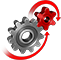# WolframSystemModeler

## COVID-19: Study Impact of Policies

During a pandemic, governments implement various policies that typically would decrease the exponential growth rate. This example shows what happens without any policy implementation and how timing is very important. The ambition is not to give any precise numbers, but to make it easier to understand how simple yet at the same time complicated it is to control a pandemic.

To run this example, you'll need

### The Model

The COVID-19 model used in this example is based on the SIR (susceptible, infectious, recovered) model. The SIR model was developed by Kermack and McKendrick in the early 1900s and is one of the most well-known models for studying infectious diseases within populations.Modified SIR model with vaccination and policy impact factor.

### Simulation

The model is simulated for an initial susceptible population of 10 million with 1 person infected. A basic reproduction number (R0) of 2.65 and mean infectious period of 7 days is used. No vaccination is considered.The model is simulated using a custom time unit of days "d". The plot has a caption that displays the values of the susceptible, infected and recovered population at the end of the simulation.

Add custom units, model plots and captions to make your plots understandable.

### Analysis

Use the locators to introduce policy measures. A policy impact factor represents the effect of measures such as lockdown and social distancing. A value of more than 1 reduces the effective reproduction number (R0hat).Observe the difference between a no-policy model and a model with policy. The dashed lines represent the results with policy. Explore the COVID-19 Policy Simulator to learn more.

Develop epidemiological models

## Wolfram System Modeler

Questions? Comments? Contact a Wolfram expert »